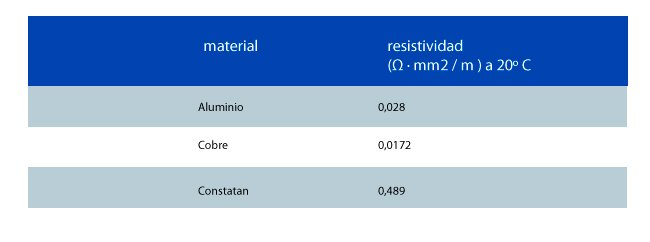Today you can find the main formulas to calculate the electrical resistance of a cable. But first let’s look at some basics on resistance.

The electrical resistance is the opposition of electrons when moving through a conductor.  Or in other words, the difficulty found by a current when traversing a closed electrical circuit, which allows slow or reduce the free flow of electrons.

According to the International System, the unit of resistance is the ohm, represented by the Greek letter omega (Ω), in honor of the German physicist Georg Ohm, who discovered that principle. Ohm is the resistance of a conductor when driving one ampere (current). Between its ends there is a potential difference (voltage) of a volt.

Firstly, to calculate the resistance of a cable it must be known the following issues:

• What is its coefficient of resistivity or specific resistance “” (rho)
• its length,
• and the section area.

For a cable, resistance is calculated by the following formula:where,

• R is the resistance in ohms (Ω).
• ρ is the resistivity in ohms-meter (Ω×m)
• l is the length of the conductor in meter (m)
• s is the cross sectional area of the conductor in square meters (m2)

In the chart below you can see the specific resistance  Ω · mm2 / m of the most common materials at a temperature of 20 ° Celsius:And here you have the formula for the area of the circumference of the conductor (surface or cross-sectional area of the material in mm2) you need to know to calculate the resistance:where,

• A is the area of the circumference of the metal part of the conductor.
• π, is the constant equal to 3.1416
• r, the radius of the circle (equivalent to half the diameter).

We hope this post has been useful for you.